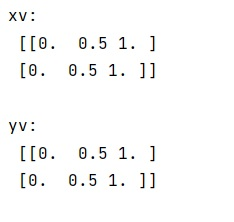# NumPy Meshgrid Function

Learn about the NumPy's Meshgrid function with examples in Python.
Submitted by Pranit Sharma, on February 11, 2023

NumPy is an abbreviated form of Numerical Python. It is used for different types of scientific operations in python. Numpy is a vast library in python which is used for almost every kind of scientific or mathematical operation. It is itself an array which is a collection of various methods and functions for processing the arrays.

## numpy.meshgrid() Method

The numpy.meshgrid() function is used to create a grid using two one-dimensional arrays.

It returns coordinate matrices from coordinate vectors. It makes N-D coordinate arrays for vectorized evaluations of N-D scalar/vector fields over N-D grids, given one-dimensional coordinate arrays x1, x2,…, xn.

This function supports both indexing conventions through the indexing keyword argument. Giving the string 'ij' returns a meshgrid with matrix indexing, while 'xy' returns a meshgrid with Cartesian indexing. In the 2-D case with inputs of length M and N, the outputs are of shape (N, M) for 'xy' indexing and (M, N) for 'ij' indexing. In the 3-D case with inputs of length M, N, and P, outputs are of shape (N, M, P) for 'xy' indexing and (M, N, P) for 'ij' indexing.

Syntax:

```numpy.meshgrid(*xi, copy=True, sparse=False, indexing='xy')
```

Parameter(s):

• x1, x2,…, xn: array_like-
Coordinates of a grid.
• Indexing: {'xy', 'ij'}, optional-
Cartesian ('xy', default) or matrix ('ij') indexing of output. See Notes for more details.

Let us understand with the help of an example,

## Python code to demonstrate the example of numpy.meshgrid() function

```# Import numpy
import numpy as np

# Creating co-ordinates
nx, ny = (3, 2)

# Matrix indexing
x = np.linspace(0, 1, nx)
y = np.linspace(0, 1, ny)

# Creating a meshgrid
xv, yv = np.meshgrid(x, y)

# Display meshgrids
print("xv:\n",xv,"\n")
print("yv:\n",xv,"\n")
```

Output: